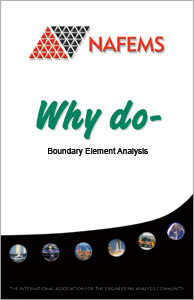# Why do Boundary Element AnalysisThe main aim of this book is to provide readers with a simple introduction to the Boundary Element method, as an alternative technique to the FE method, without resorting to complex mathematical theory and jargon. Throughout the booklet, emphasis will be placed on the physical significance of the Boundary Element theory rather than its mathematical details. The coverage of the Boundary Element method in this booklet is limited to linear static analysis, with a brief introduction to non-linear problems. Sufficient references are given to enable readers to seek further details of Boundary Element formulations.

This booklet is aimed at readers who are reasonably familiar with the FE method and its application to engineering problems. It is assumed that the reader is a graduate in any engineering discipline, applied mathematics or physics with no previous knowledge of the mathematical foundation of Boundary Element methods.

## Contents

### 1. Introduction

• 1.1 What is the Boundary Element method?
• 1.2 Scope
• 1.4 Layout

### 2. A brief History of the BE Method

• 3. Comparison of BE and FE Methods
• 3.1 Advantages of the BE Method
• 3.2 Disadvantages of the BE Method
• 3.3 Suitability of the BE Method

### 5. Theoretical foundation of the Boundary Element Method

• 5.1 The Differential Equations of Displacements
• 5.2 The Point Load Solution
• 5.3 The Reciprocal Work Theorem
• 5.4 The boundary integral equation

### 6. Implementing Boundary Element Formulation on a computer

• 6.1 Division of the Surface into Boundary Elements
• 6.2 Numerical Integration
• 6.3 Formation of Linear Algebraic Equations
• 6.4 Application of the Boundary Conditions
• 6.5 Solving the Equations
• 6.6 Computing Internal Displacements and Stresses

### 7. Dealing with Non-linear Problems

• 7.1 BE Analytical Non-Linear Formulations
• 7.2 BE Numerical Non-Linear Implementation

### 8. Some Examples of BE Applications

• 8.1 Design of a Pressure Vessel Door Closure
• 8.2 BE Analysis of Fracture Problems
• 8.3 BE Analysis of Contact Stresses in a Pressure Vessel
• 8.4 Analysis of Threaded Connections
• 8.5 Analysis of Thermal Stresses
• 8.6 BE Analysis of Plasticity in a Perforated Plate
• 8.7 BE Analysis of 3D contact Problems
• 8.8 BE Analysis of Inverse Stress Analysis Problems

### 9. Concluding Remarks

References

Bibliography - A Selection of BE Textbooks

### Product Details

Order Ref: HT29

Member Price
£17.50 | \$21.73 | €19.38
Non-member Price
£75.00 | \$93.11 | €83.02